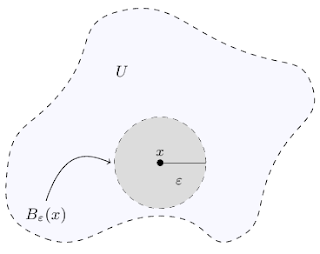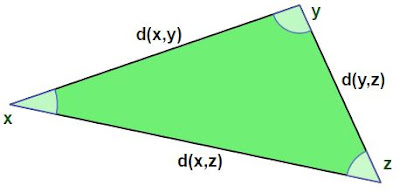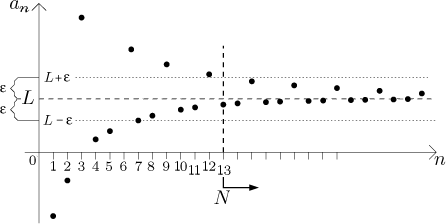## How close is "close enough"? Metric Spaces, Topological Spaces, and Convergence

Preliminaries: set basics, Euclidean space

### Open and closed sets; topology

You may be familiar with the concept of open and closed sets in Euclidean space ${\Bbb R}^n$. For those who aren’t, an open set is one that does not contain any of its boundary points. A closed set is one that contains all of its boundary points. The following graphic provides a good illustration (dotted lines indicate boundary points not included in the set, while solid lines indicate boundary points which are included):Diagram 1: an intuitive depiction of open and closed sets

The complement of an open set is closed, and the complement of a closed set is open. The top-left item in the diagram also shows that some sets are neither open nor closed. In addition, the entire space ${\Bbb R}^n$ is both open and closed, as is the empty set.

Furthermore, taking unions and intersections of open and closed sets yield the following results, best summarized in a diagram:Diagram 2: unions and intersections of open and closed sets

The following definition formalizes the notion of an open set in ${\Bbb R}^n$ and allows us to justify the conclusions above.

Definition of open/closed sets in ${\Bbb R}^n$: An open set is a set $U$ such that for each $x \in U$, there exists an $\epsilon>0$ (which may be very small) such that $y \in U$ whenever the distance between $x$ and $y$ is less than $\epsilon$. A closed set is one whose complement is an open set.Diagram 3: definition of an open set $U$ in the plane ${\Bbb R}^2$

The set of all points $y$ located strictly within distance $\epsilon$ of a point $x$ is called the open ball of radius $\epsilon$ centered at $x$ and is denoted $B_{\epsilon}(x)$ as shown in the picture. Using this definition of open sets, it is easy to prove the properties in Diagram 2.

Note that the definition presented above actually relied on our having a notion of distance between two points. In a general space, this may not be the case, or there may be numerous measures of distance from which we can choose. The field of topology studies properties of more general spaces without necessarily relying on a distance function. To start, we define the open sets as a collection having some of the familiar properties presented above.

Definition of a topology: Let $X$ be a set, and let $\mathscr T$ be a subset of the power set of $X$, i.e. a collection of subsets of $X$. $\mathscr T$ is called a topology on $X$, and the elements of $\mathscr T$ are called open sets, if the following properties hold:
1. The empty set and $X$ itself are in $\mathscr T$.
2. Any union of (finitely or infinitely many) elements of $\mathscr T$ is also an element of $\mathscr T$.
3. Any intersection of finitely many elements of $\mathscr T$ is also an element of $\mathscr T$.
Closed sets are defined as sets whose complements are open sets.

The "dumbest" example of a topology on a set $X$ is the set $\{ X , \emptyset \}$, called the discreet topology. Another example for $X = {\Bbb R}^n$ was the above-mentioned usual definition of open sets using the distance function. Namely, the open sets in ${\Bbb R}^n$ (in the usual topology) are the ones which contain an open ball of some radius around each of their points, or equivalently, contain none of their boundary points.

The open sets defined via open balls as described above comprise the so-called metric topology, in which every open set can be written as the union of open balls, and every point is contained in an open ball (actually, infinitely many open balls). Because of these two facts, we say that the open balls form a base for, or generate, the metric topology.

### Metric Spaces

In defining the above-described metric topology on ${\Bbb R}^n$, we made use of the fact that we can measure distance between two points using the well-known distance formula. Similarly to the way we used the properties from ${\Bbb R}^n$ as the definitions of topological spaces and vector spaces, we can do the same to define the properties a distance measure should have.

A distance function on a set $X$ should map any two elements of $X$ to a "distance." Formally, a function $d: X \times X \rightarrow [0, \infty)$ (i.e. a function taking any two inputs from $X$ and returning a non-negative real number) is called a metric if it satisfies the following 3 properties:

1. Positive-definiteness: $d(x,y) \geq 0$, with $d(x,y)=0 \iff x=y$,
2. Symmetry: $d(x,y)=d(y,x)$, and
3. Triangle inequality: $d(x,z) \leq d(x,y) + d(y,z)$.The triangle inequality: the length of one side of a triangle cannot exceed the sum of the lengths of the other two.

For example, on the real number line, the absolute value of the difference between two numbers (i.e. the distance formula in one dimension) is a metric. The symmetry and positive-definiteness of $d(x,y) = |y-x|$ are obvious; to prove the triangle inequality $|z-x| \leq |z-y| + |y-x|$ for all real numbers $x,y,z$, note that we can set $a = z-y$ and $b=y-x$ so that $z-x = a+b$. Thus it suffices to prove that $|a+b| \leq |a| + |b|$ for all $a,b \in {\Bbb R}$.

Proof of the triangle inequality for absolute value: Note that \begin{align} -|a| &\leq a \leq |a| \tag{1} \\ -|b| &\leq b \leq |b| \tag{2} \end{align} Adding equations (1) and (2) gives $$-(|a|+|b|) \leq a+b \leq |a|+|b| \tag{\star}$$ To simplify the notation, call $a+b := \phi$ and call $|a|+|b| := \psi$. Then equation $( \star )$ says that $-\psi \leq \phi \leq \psi$. In other words, $| \phi | \leq \psi$. Translating back to $a$ and $b$, we have shown that $|a+b| \leq |a| + |b|$.
$\square$

So the usual distance measure on the real number line is indeed a metric, making ${\Bbb R}$ a metric space. The usual topology on ${\Bbb R}$ is the metric topology generated by the open intervals $(a,b) = \{ x \ | \ a<x<b \}$. These are also the open balls defined by the absolute value metric, since $(a,b) = B_{(b-a)/2}(\frac{a+b}{2})$ and $B_{\epsilon}(x) = (x-\epsilon, x+\epsilon)$.

Given a topological space $(X, {\mathscr T})$, if the elements of $\mathscr T$, i.e. the open sets, are generated by the open balls $B_{\epsilon}(x)$ in some metric, then $(X, {\mathscr T})$ is called metrizable. There are examples of non-metrizable topological spaces which arise in practice, but in the interest of a reasonable post length, I will defer presenting any such examples until the next post. However, it is worth noting that non-metrizable spaces are the ones which necessitate the study of topology independent of any metric.

### Convergence of sequences in a metric space

Suppose we have a sequence of points $x_1, x_2, x_3, \dotsc$ in a metric space $(X,d)$. To simplify the notation, such a sequence is often written as $(x_n)$. How do we know if the sequence has a limit? Take a look at the following diagrams:

The first sequence of points obviously does not have a limit; it never "closes in" on any point. The second sequence, on the other hand, does seem to have a limit. The formal definition makes this intuition concrete.

Definition of limit of a sequence (metric space): Let $(x_n)$ be a sequence in the metric space $(X,d)$. The point $L \in X$ is called the limit of $(x_n)$ if, for any $\epsilon >0$, there exists a number $N$ such that $d(x_n, L) < \epsilon$ whenever $n>N$. In this case, we write $L = \displaystyle{\lim_{n \rightarrow \infty}{x_n}}$.
$\square$

This means that given any threshold $\epsilon$, which can be arbitrarily small, the sequence points are eventually (i.e. for $n>N$, where $N$ may depend on $\epsilon$ and may be very large) all within distance $\epsilon$ of $L$.Illustration of a convergent sequence: here, the sequence terms are all within $\epsilon$ of $L$ after the 13th term. So for the choice of $\epsilon$ shown, $N=13$ works.

For example, the sequence of real numbers $1, \frac{1}{2}, \frac{1}{4}, \frac{1}{8}, \dotsc, \frac{1}{2^n}, \dotsc$ has limit $0$: take a very small tolerance such as $\epsilon = \frac{1}{1 \mathord{,} 000 \mathord{,} 000}$. Then we have
$$\left| \frac{1}{2^n} - 0 \right| < \frac{1}{1 \mathord{,} 000 \mathord{,} 000} \iff 2^n > 1 \mathord{,} 000 \mathord{,} 000 \iff n > \log_{2}{1 \mathord{,} 000 \mathord{,} 000}$$Similarly, for a general $\epsilon$, we have $|x_n-0| < \epsilon$ whenever $n$ is larger than $N = \log_{2}{(1 / \epsilon)}$. Since we have found a suitable $N$ for any $\epsilon$, $0$ is indeed the limit of the sequence.

### Convergence of sequences in a topological space

A topology $\mathscr T$ on a set $X$ specifies open and closed sets independently of any metric which may or may not exist on $X$. The definition of convergence above relied on a metric, but we can remove this reliance by defining convergence purely in terms of open sets.

Definition of limit of a sequence (topological space): Let $(x_n)$ be a sequence in the topological space $(X, {\mathscr T})$. The point $L \in X$ is called the limit of $(x_n)$ if, for any open set $U$ containing $L$, there exists a number $N$ such that $x_n \in U$ whenever $n>N$. In this case, we write $L = \displaystyle{\lim_{n \rightarrow \infty}{x_n}}$.
$\square$

In the metric space case above, these open sets $U$ were simply the open balls $B_{\epsilon}(L)$, so the two definitions agree in a metrizable space. Without a metric by which to define open balls in a general topological space, we replace these with open sets $U$ containing $L$, known as neighborhoods of $L$.

To conclude this post, let's take a look at an easy proof that demonstrates how things are done in topology and also ties in the notion of convergence.

Recall that the closed sets in ${\Bbb R}^n$ are the ones containing all of their boundary points, or equivalently, the limits of all their convergent sequences (can you picture why every boundary point, as well as every interior point, of a set is the limit of a convergent sequence contained in that set?). An analogous statement holds in general topological spaces.

Closed sets contain their limit points: let $(X, {\mathscr T})$ be a topological space, and let $C \subseteq X$ be a closed set. Let $(c_n)$ be a convergent sequence of points all contained in $C$, with $\displaystyle{\lim_{n \rightarrow \infty}{c_n}}=L$. Then $L \in C$.

Proof: Since $C$ is closed, its complement $X-C$ is open (by the definition of closed sets). Assume $L \notin C$. Then $L \in X - C$, an open set. Since $X-C$ is an open set containing $L$, the definition of convergence tells us that there exists an $N$ such that $c_n \in X-C$ for all $n > N$. But this is a contradiction, since $c_n \in C$ for all $n$.
$\square$

In the next post, we'll explore a few topologies on sets of functions, and we'll see that the most intuitive one is not metrizable.

I hope you enjoyed this post, and please feel free to post any questions in the comments section below.

## Sets of Functions

This post will introduce sets whose elements are functions and explain the notation for these sets. If you aren't familiar with basic notations for sets, please take a look at this post.

Suppose $f$ is a function mapping inputs from a set $X$ (the domain of $f$) to outputs (called values) in a set $Y$ (the range of $f$). Note that each input is mapped to exactly one output (this is the definition of a function), but numerous inputs are allowed map to one output value. We record this relationship by writing $f : X \rightarrow Y$.An example of a function; in this case, two inputs map to a single output.

### The set of all functions $X \rightarrow Y$

Recall that the Cartesian product of two sets $A$ and $B$, denoted $A \times B$, is the set of ordered pairs $(a,b)$ where $a \in A$ and $b \in B$. In particular, the Cartesian product of a set $Y$ with itself, $Y \times Y$, is the set of ordered pairs $(y_1, y_2)$ where both $y_1$ and $y_2$ are elements of $Y$.

Extending this a bit further, for a positive integer $n$, we can write $Y^{n}$ (also written $\underbrace{Y \times Y \times \dotsb \times Y}_{n \rm \ times}$ or $\prod_{i=1}^{n}{Y}$) to denote the set of ordered $n$-tuples $(y_1, y_2, \dotsc , y_n)$ where all of the $y_i$'s are elements of $Y$.

Suppose $X$ is a finite set with $|X|=n$ (i.e. $X$ has $n$ elements), $\{ x_1, x_2, \dotsc, x_n \}$. If we have some function $f: X \rightarrow Y$, then $f(x_{1})$ will be some element $y_1$ of $Y$, $f(x_{2})$ will be some element $y_2$ of $Y$, etc. Therefore, specifying the function amounts to specifying an $n$-tuple of elements of $Y$, $(y_1, y_2, \dotsc, y_n)$, one for each input from the domain $X$. In other words, specifying $f$ amounts to specifying an element of $Y^n$.

Similarly, any $n$-tuple $(y_1, y_2, \dotsc , y_n)$ from $Y^n$ gives us a function from $X$ (or any other set with $n$ elements, such as $\{ 1, 2, \dotsc , n \}$), namely \begin{align} f(x_1) &= y_1 \\ f(x_2) &= y_2 \\ & \vdots \\ f(x_n) &= y_n \end{align} Thus we can identify functions $X \rightarrow Y$ one-to-one with elements of $Y^n$ if $|X|=n$. For this reason, we use the notation $Y^X$ for the set of all functions $X \rightarrow Y$.

This notation is also used when $X$ is not a finite set. For example, if $X = {\Bbb N}$, the set of natural numbers $\{ 1,2,3, \dotsc \}$ and $Y={\Bbb R}$, the real numbers, then functions ${\Bbb N} \rightarrow {\Bbb R}$ can be viewed as sequences of real numbers $(r_1, r_2, r_3, \dotsc)$. By the same logic as above, we can view ${\Bbb R}^{\Bbb N}$ as the Cartesian product $\prod_{n \in {\Bbb N}}{{\Bbb R}} = {\Bbb R} \times {\Bbb R} \times {\Bbb R} \times \dotsb$.

Analogously, we use the same notation when $X$ is not even countably infinite, e.g. $X = {\Bbb R}$, the real numbers. The set ${\Bbb R}^{\Bbb R}$ of all functions ${\Bbb R} \rightarrow {\Bbb R}$ can be thought of as the set of "vectors" with one component for each real number $x \in {\Bbb R}$. There are too many real numbers to write these out as tuple as we could in the finite and countably infinite cases above, but we can still write a Cartesian product of an uncountable infinity of copies of ${\Bbb R}$, and thus we write the set of all functions as ${\Bbb R}^{\Bbb R}$, identifying it with $\prod_{x \in {\Bbb R}}{{\Bbb R}}$.

The next few posts will show how we can define a vector space structure and a notion of convergence of a sequence of functions (i.e. a sequence of points in $Y^X$), extending our intuition from vectors in ${\Bbb R}^n$. We need this rigorous definition of convergence of functions to a limit function in order to develop models of random phenomena in physics, finance, etc. using stochastic processes.